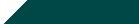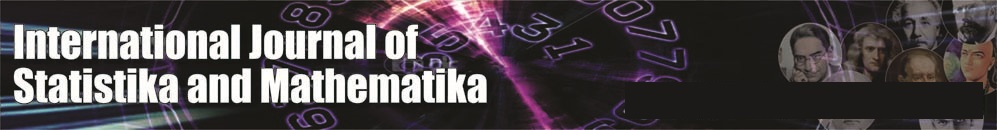``Home| Journals | Statistics Online Expert | About Us | Contact UsUntitled Document

An Inventory Model for Weibull Deteriorating Items with Linear Demand and Permissible Delay in Payments under Inflation

Sushil Kumar*, U. S. Rajput

Department of Mathematics and Astronomy, University of Lucknow, Lucknow, Uttar Pradesh-226007 INDIA.

[email protected]

Research Article

Abstract: This paper proposes an optimal replenishment policy for Weibull deteriorating items with a permissible delay in payment under inflation over a finite planning horizon and the demand rate is a linear function of time.

Keywords: Inventory, Inflation, Weibull-distribution, Permissible delay in payments.

Introduction

Many Mathematical models have been developed for controlling inventory and in the earlier models many researchers consider the constant demand rate which is a feature of static environment while the dynamic environment nothing is fixed or constant. So in most of the cases the demand for items increases with time. But most of the organizations are working towards increasing demand of their items with time. Basically the items that are stored for future use always loose part of their value with passage of time. In inventory this phenomenon is known as deterioration of items. The rate of deterioration is very small in some items like hardware, glassware, toys and steel. The items such as medicine, vegetables, gasoline alcohol, radioactive chemicals and food grains deteriorate rapidly overtime so the effect of deterioration of physical goods cannot be disregarded in many inventory systems.               The deterioration of goods is a realistic phenomenon in many inventory systems. The controlling of deteriorating items becomes a measure problem in any inventory system. The researchers have continuously modified the deteriorating inventory models so as to become more practicable and realistic. Initially Chare & Schrader  consider an inventory model with a constant rate of decay. Covert and Philip  extended Ghare and Schrader's model for variable rate of deterioration by assuming Weibull's two parameter distribution function. Later these models are modified by Philip , Mishra , Chakrabarty, Giri and Choudhary . Hence the realistic models treat with a time varying deterioration rate. In the models mentioned above the inflation and time value of money have no significant role with inventory policy. It is realized that most of the countries are suffering from high inflation that leads decline of time value of money. Hence in many inventory models ignorance of inflation and time value of money is disregarded. Buzacott  developed an inventory model with inflation, several researchers developed their model by considering various inflation rate are time value of money. Bierman and thomas , Chandra and Bahner , Gor and Shah  Kuolung Huo , Mishra , Moon and Giri  developed the value able models by considering various inflation rate. In the traditional EOQ models the purchaser pays for his items as soon as he received the items but in business organizations the supplier may allow a credit period to encourage the customers delay in payment by the purchaser to the supplier in an alternate way of price discount. The valuable models in this regards are given by Goyal , Lio . It is empirically observed that the failure and life expectancy of many items can be expressed in terms of Weibull distribution function.

In the present paper we consider a Weibull distribution function of two parameters for deterioration. The demand rate is considered as a linear function of time and solves the model analytically by numerical examples.

Notations and Assumptions:- We consider the following assumptions

H                     =          Length of finite planning Horizon.

R                     =          (a+bt), Demand Rate

p                      =          Selling price per unit at time t = o

P(t)                 =          perT, Instantaneous selling price per unit.

C(t)                 =          cerT , Instantaneous ordering cost per order.

Ie                    =          Interest earned per annum by the Retailer.

Ic                     =          Interest charged in stock per annum by the supplier.

T1                    =          Allowable delay period during settlement of the account.

Q                     =          Optimum ordering quantity.

T                      =          Optimum length of cycle time.

(t)                 =          abtb-1 ,Weibull distribution, 0<a<1, b>1.

r                      =          inflation rate.

B                     =

I (t)                 =          Instantaneous inventory level

TC (T)             =          Total relevant cost over [O,H]

NP (T)             =          Total relevant profit over finite planning horizon.

Oc                   =          Ordering cost

Dc                   =          Deterioration cost

Hc                   =          Holding cost

Ic                     =          Interest charged for unsold items at initial time.

IE                     =          Interest earned from sales during the permission

delay period [o, T1].

Hence total cost TC (T)     =              OC + DC + HC + IC - IE

and the gross revenue                      =              R(P-C) erT

Assumptions:-

1. Inflation rate is constant.
2. Shortages are not allowed
4. No repair or replacement of deteriorated items during the cycle.
5. At the end of trade credit period the customer pay off all units ordered and begins paying for the interest charged on the items in stock.

Mathematical Formulation

Let H=nT, where n is the number of replenishments occur during the period H, be the planning horizon. Then the differential equation of instantaneous level of inventory I(t) is given by,

…… (1)

With boundary conditions

Then the solution of (1) is

……… (2)

Using the initial condition I (o) = Q, Then the initial ordering of quantity is

Q= ………. .(3)

The ordering cost or set up cost is given by

Oc = A (o) + A (T) + A (2T) + ……..+ A {(n-1)T}

Using A (t) = A erT, then

Oc = A + A erT + A2rT+------+ Ae (n-1) rT

,   where H = nT

, where B = erH -1

Thus

Oc   -------- (4)

The deterioration cost is given by

Dc = I (o) – RT

Dc =

DC = ----- (5)

The inventory holding cost is given by

Hc =

Hc = …...(6)

Depending upon the cycle time T and customer’s choice the    following two cases arise

Case I: - Interest charged in [O, H]

Since in [O, H], the cycle length T is greater than the permissible delay time T1,

Then the interest charged in [T1, T] is

IC1 =

=

---------- (7)

The Interest earned in [0, H] is given by

IE1 = Ie

IE1 = …….. (8)

The Average cost: - The total average cost per unit in the interval [o, H] is,

TC (T) = …… (9)

Net Profit: - The net profit in the interval [o, H] is given by

NP1 (T) = R (P-C)erT -TC (T)

NP1 (T) = [(a+ bt) (P-C)erT- TC (T)]………..(10)

For optimality condition of total average cost: - We have

Now find separately ….. etc.

So  ….. (11)

…….. (12)

Since

…………..(13)

+ ……….(14)

Then

………….(15)

+

+ ……………(16)

+

………(17)

+ ………..(18)

Then

Similarly

Now

And,  gives the optimal condition for net profit

So,  gives the sufficient condition for net profit at  where  is the solution of

Case II –Interest earned:-If the optimal cycle length T is less than the permissible delay time then the interest earned during [0, H] is given by  so

=   +

………..(18)

And

…….(19)

So

Numerical problem

Let A = 100, a = 10, b = 5, C = 20, P = 30, r = 0.01

= 0.05, year, h = 0.03, Ie = 0.10, Ic = 0.15,

, ,

At, t = 0, Then the total cost is TC (T) = 53317.035

Numerical problem:- Let A = 100, a = 10, b = 5, C = 20, P = 30, r = 0.0125

= 0.0625, year, h = 0.03, Ie = 0.10, Ic = 0.15,

, ,

And t = 0, Then the total cost is TC (T) = 256841.0801

Conclusion

Under Constant Weibull deterioration, keeping the parameters, optimum cycle time T and allowable credit period T1 fixed and increase in parameter r (inflation rate) increases the total cost. Hence the model becomes more profitable and very useful in business organizations dealing with perishable products, domestic goods and other products.

References

1. Bierman, H and Thomas. J. (1977):- Inventory decisions under inflation Condition Decision Science, 8, 151-155.
2. Buzacott, J.A. (1975):- Economic order quantities with inflation, OR Quarterly, 26, 553-558.
3. Chandra, M.J. and Bahner, M.J. (1985):- The effect of inflation and time value of many on some inventory systems, international Journal of production research, 23, 723-729.
4. Chakrabarty, T, B.C. Giri and K.S. Chaudhari (1998):- An EOQ model for items with Weibull distribution deterioration shortages and trended demand. 649- 657.
5. Covert, R.P. and Philip G.C. (1973):- An EOQ model for items with Weibull distribution deterioration. AIIE Transaction, 5, 323-326.
6. Ghare, P.M. and Schrader, G.H. (1963):- A model for exponential decaying inventory system, Journal of industrial Engineering, 15, 238-243.
7. Gor, R. and Shah, N.H. (2006):- An EOQ model for deteriorating items with price dependent demand and permissible delay in payments under inflation, 43, 4, 377-388.
8. Goyal, S.K. (1985):- EOQ under conditions of permissible delay in payments, Journal or Society, 24(6), 539-547.
9. Kuo-Lung Hou (2006):- An inventory model for deteriorating items with stock dependent consumption for deteriorating items with stock dependent consumption rate and shortages under inflation and time discounting, European Journal of O.R. 168, 463-474.
10. Lio, H.C.C.H. Tsai and C.T. su (2006):- An inventory models with deteriorating items under inflation when a delay in payment is permissible, International Journal of production Economics, 63, 207-214.
11. Mishra, R.B. (1975):- Optimum production lot size model for a system with deteriorating inventory. International Journal of production Research, 13, 495-505.
12. Mishra, R.B. (1979):- A study of inflation effects on inventory system Logistics spectrum, 9, 260-268.
13. Moon, I, Giri, B.C. and Ko,B(2005):- EOQ models for ameliorating deteriorating items under inflation and time discoursing EJOR. 162, 773-785.
14. Philip, G.C. (1974):- A generalized EOQ model for items with Weibull distribution deterioration AIIE transaction, 6, 159-162.
15. V.K. Mishra, S.K. Sahu, Bhaskar Bhaula & L.K. Raju (2010):- An inventory model for Weibull deteriorating items with permissible delay in payments under inflation IJRRAS, 6-1-02.